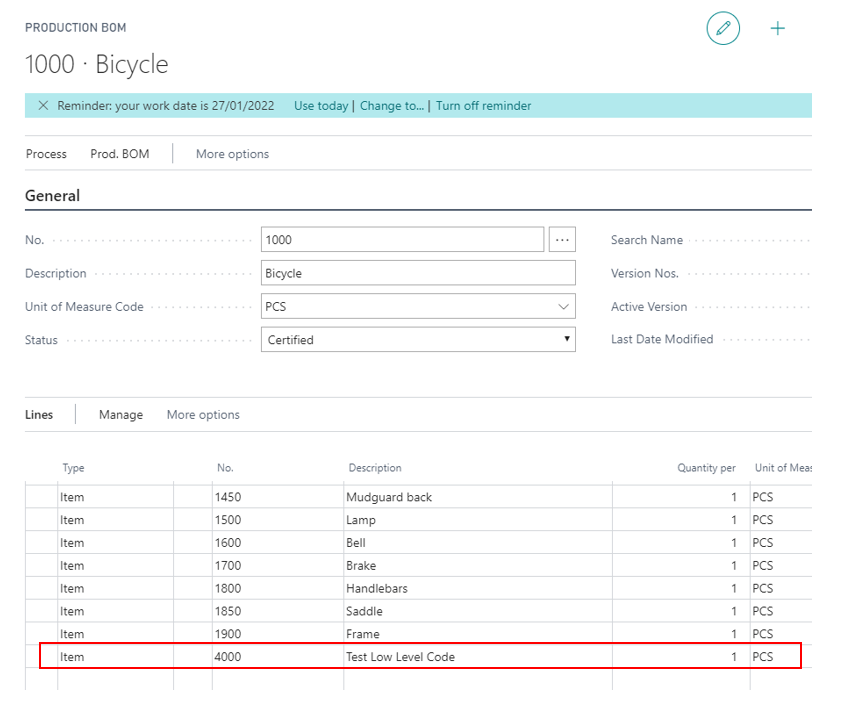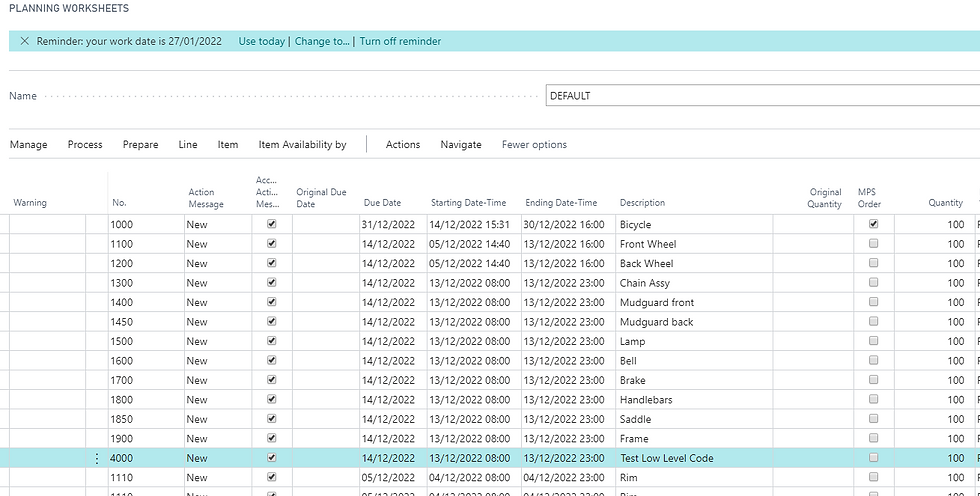top of page
Cerca
• marco ferrari

# How a wrong Low-Level Code can affect planning calculation

This post is in some ways similar to the one about garbage in MRP.

I would like to show how a wrong value into the Low-Level Code field can affect the calculation in the MRP.

First of all, let's open the Manufacturing Setup and disable the field Dynamic Low-Level Code. This switch tells the system to calculate the Low-Level Code field into the Item table every time you set the BOM Status to Certified.If you use this setup, changing the BOM status is faster, but before running the MRP you have to remember to run the Calculate Low-Level Code task.

But, what is the Low-Level Code and why is it so important?

Suppose to have this BOMAs you can see, Item B appears at level 1 and level 3; ok, the Low-Level Code for this item is equal to 3. So this field represents the lowest possible level in which an item is contained in a BOM.

Why is this information so important during the MRP? This is due to the fact that the planning engine reads the items exactly by this field, as you can see below:If you run the MPS/MRP for the structure above, the sequence will be:

1. Item A (MPS)

2. Item C

3. Item D

4. Item E

5. Item F

6. Item G

7. Item B

This is done simply bacause the net requirement of an item at level n depends on the net requirement at level (n - 1). For the BOM above, you cannot know how much quantity you need of item B (level 3), if you don't know the needed quantity of G (level 2), and so on. That's why calculation for item B must be done at the end.

Now for our purpose let's create the Item 4000 - Test Low Level Code, and let's put it in the bicycle's production BOMIf you run the Calculate Low-Level Code task you can see that 1 appears in the Low-Level Code field (I've added the field on the Item Card).If I run the MRP I can see this result:Now I add Item 4000 into the production BOM of item 1250 and I forget to calculate the low-level code that should change to 3.Now the net requirement for item 4000 depends not only on the bicycle, but on the Hub too. The system does not see this: if I run the MRP I cannot see any change in the calculation:If I run the Calculate Low-Level Code task and the MRP, I can see the correct calculation:Now you can understand why this field is so important when you run the MRP. This information cannot be wrong, and if you're not sure is correct or the calculation of Low level-Code stops with an error because "the bicycle appears in the bicycle's BOM", it's completely self-defeating to run the MRP in this scenario. Don't do until you made a review of your BOMs and run the task.

PS: please note that activating the dynamic calculation in the Manufacturing Setup table does not guarantee that the Low-Level Code is always correct: when you remove an item in a BOM, the certification does not recalculate the new level for the item you removed. So consider to run the calculation periodically in any case.

127 visualizzazioni0 commenti
bottom of page